# Implementation

## Writing Python Wrapper for Bioinformatics Tools

The use of computational tools for data preprocessing and analysis is daily activity for any bioinformatician. Having a interdisciplinary field, a plethora of tools exist in the domain, written by developers from various fields and languages. Many times there can be multiple candidate tools exists to accomplish any given task. Often it is the decision …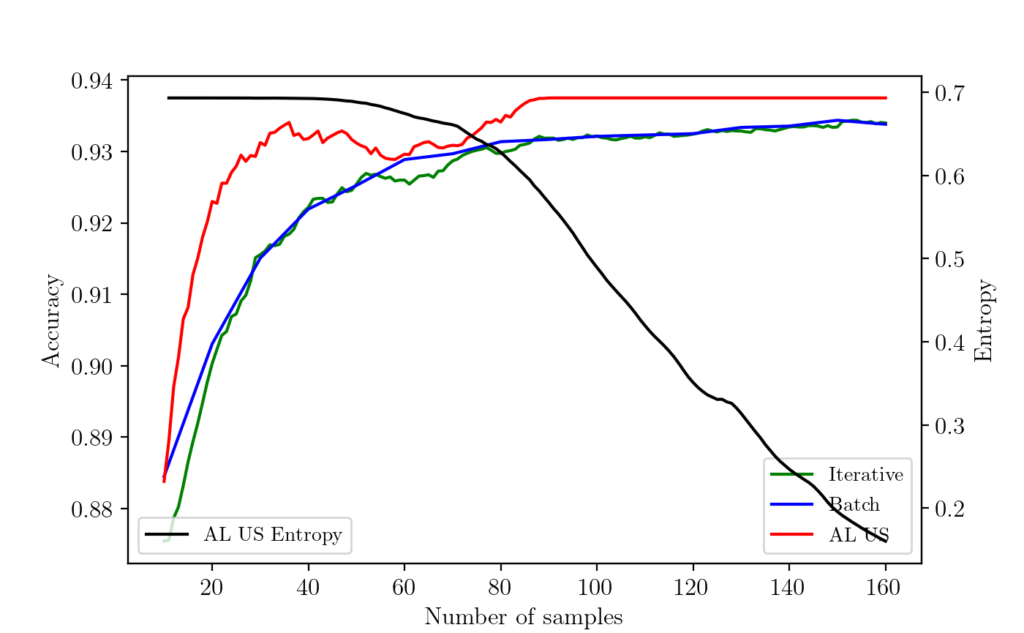## Active Learning with Uncertainty Sampling from Scratch

This article is a tutorial on the algorithm called active Learning with uncertainty Sampling. Introduction Availability of mass quantities of digital data and feasible computing power brought to the creation of learning algorithms. These learning algorithms have been benchmarked to perform specialized tasks such as classification, object detection, image segmentation, etc. The key assumption here …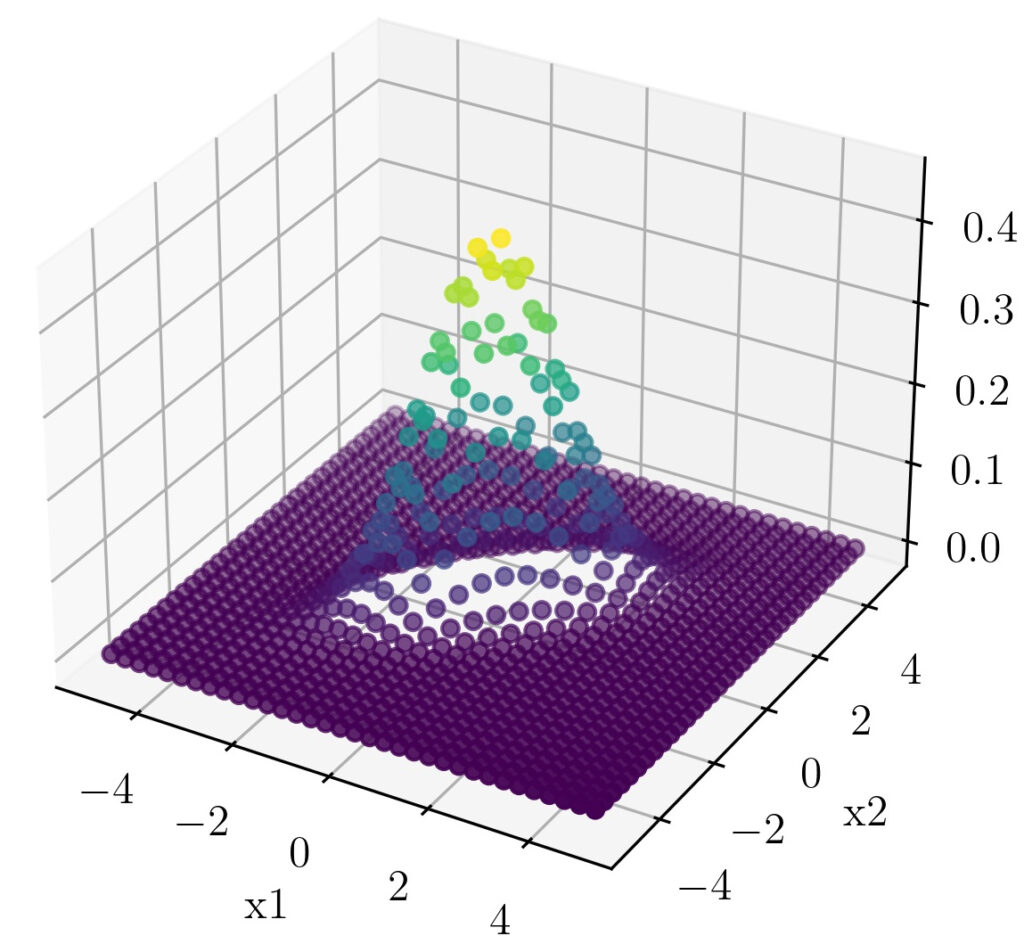## Hacking the Bivariate Gaussian Distribution

In one of our earlier posts, we have seen how we can visually relate the parts of the one-dimensional Gaussian distribution equation. In this post, we will follow the same strategy to understand the terms that comes up with a Multivariable Gaussian distribution. We will focus on the Bivariate Gaussian distribution as distributions of higher-order …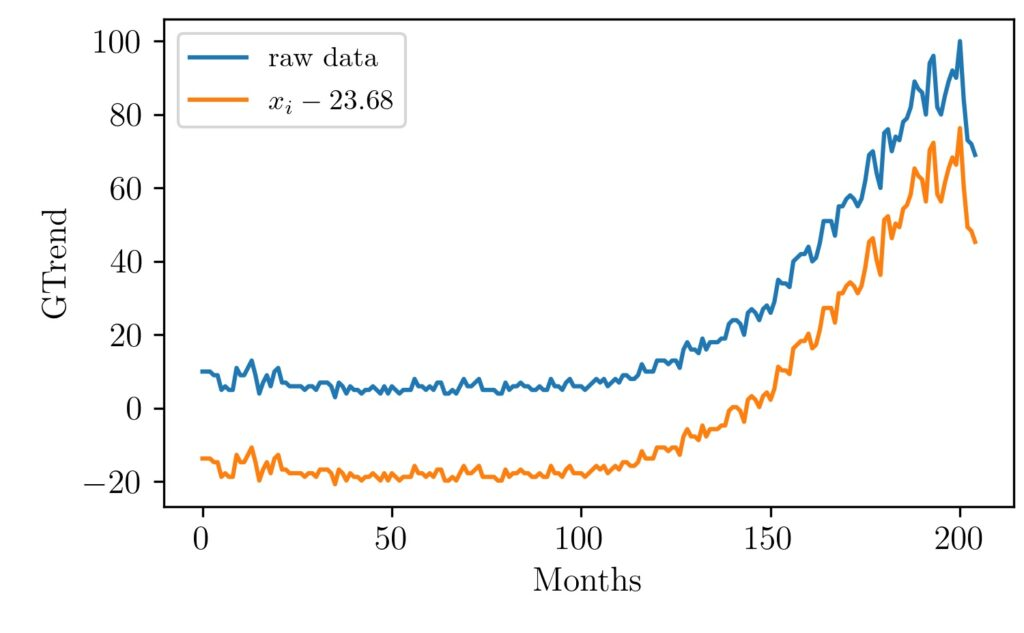## Visualizing MinMax Scaling

This article explains the minmax scaling operation using visual examples. Normalization of vectors, an array of values, signals is often used as a preprocessing step before many algorithms. For example, in machine learning, some types of algorithms are prone to different inherent scales of features. In such situations normalization is done to give the same …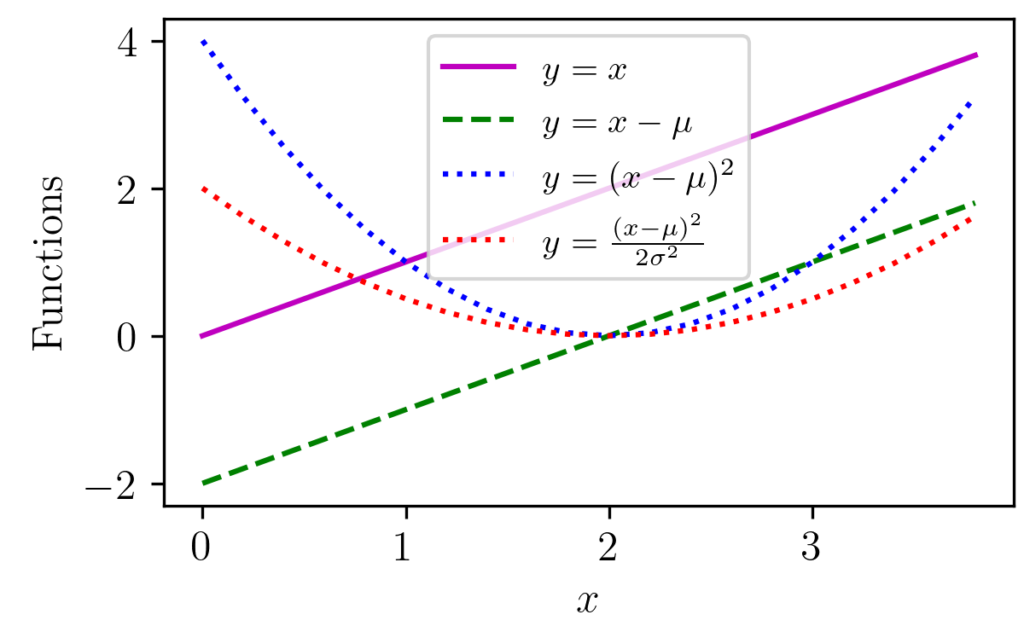## Gaussian Distribution Explained Visually

Gaussian distribution appears in various parts of science and engineering. Apart from a distribution often appear in nature, it has got important properties such as its relation to Central Limit Theorem (CLT). The figure above shows one-dimensional Gaussian distributions of various mean and variance values. Libraries like NumPy provide functions that can return Gaussian distribution …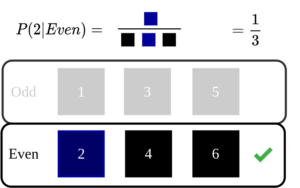## An Intuitive Explanation of Naive Bayes Classifier

Introduction In this post, let’s take a look at the intuition behind Naive Bayes Classifier used in machine learning. Naive Bayes classifier is one of the basic algorithms often encountered in machine learning applications. If linear regression was based on concepts from linear algebra and calculus, naive Bayes classifier mostly backed up by probability theory. …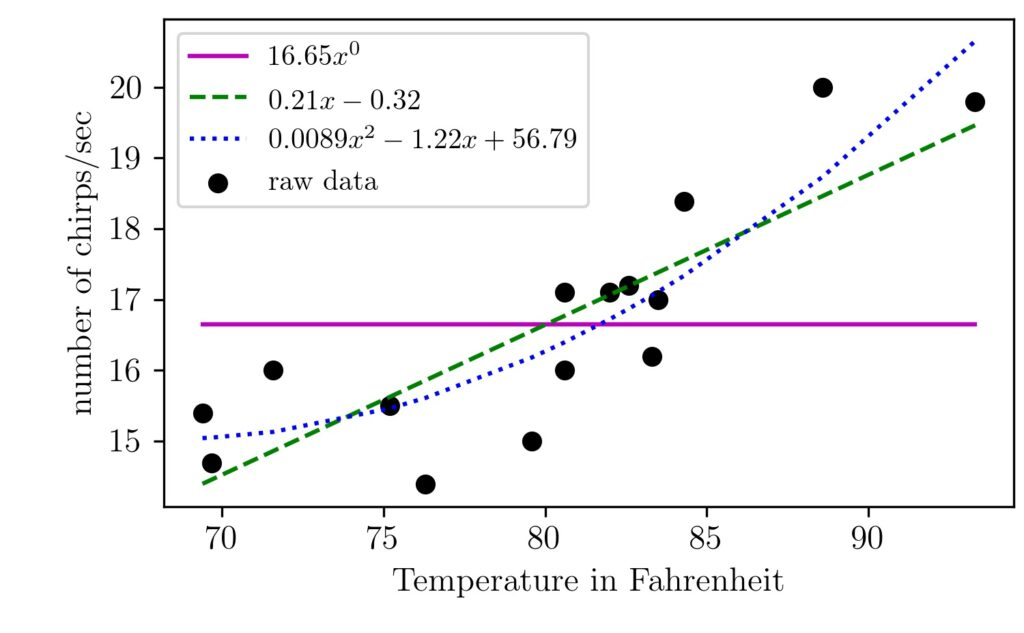## An Intuitive Explanation of Linear Regression

Linear regression is one of the algorithm machine learning enthusiasts start to learn first. In this article, l will walk you through the linear regression intuition. We will implement the basic form of it without using any machine learning packages. The main philosophy of supervised machine learning algorithms is to learn from data. The algorithms …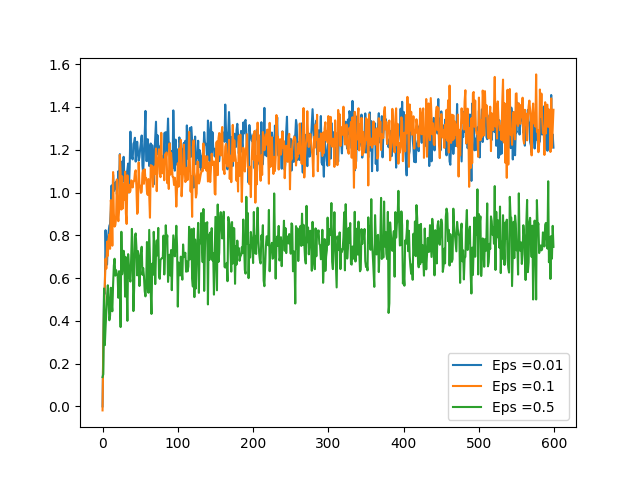## Epsilon Greedy Algorithm in Bandit Problems

Introduction Bandit problems are the simplest possible reinforcement learning scenario. Here the bandit machine can have k arms and pulling each arm leaves the user a reward. One of the arms will be giving higher rewards in the long run and moreover this pattern could be changing over a time period. Think of the scenario …